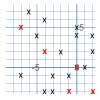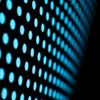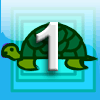# Resources tagged with: Squares

Filter by: Content type:
Age range:
Challenge level:

There are 39 NRICH Mathematical resources connected to Squares, you may find related items under Angles, Polygons, and Geometrical Proof.

Broad Topics > Angles, Polygons, and Geometrical Proof > Squares### Vector Journeys

##### Age 14 to 18Challenge Level

Charlie likes to go for walks around a square park, while Alison likes to cut across diagonally. Can you find relationships between the vectors they walk along?### Baravelle

##### Age 7 to 16Challenge Level

What can you see? What do you notice? What questions can you ask?### Eight Hidden Squares

##### Age 7 to 14Challenge Level

On the graph there are 28 marked points. These points all mark the vertices (corners) of eight hidden squares. Can you find the eight hidden squares?### Squares in Rectangles

##### Age 11 to 14Challenge Level

A 2 by 3 rectangle contains 8 squares and a 3 by 4 rectangle contains 20 squares. What size rectangle(s) contain(s) exactly 100 squares? Can you find them all?### Square Coordinates

##### Age 11 to 14Challenge Level

A tilted square is a square with no horizontal sides. Can you devise a general instruction for the construction of a square when you are given just one of its sides?### Ten Hidden Squares

##### Age 7 to 14Challenge Level

These points all mark the vertices (corners) of ten hidden squares. Can you find the 10 hidden squares?### Square It

##### Age 11 to 16Challenge Level

Players take it in turns to choose a dot on the grid. The winner is the first to have four dots that can be joined to form a square.### On the Edge

##### Age 11 to 14Challenge Level

If you move the tiles around, can you make squares with different coloured edges?### Semi-detached

##### Age 14 to 16Challenge Level

A square of area 40 square cms is inscribed in a semicircle. Find the area of the square that could be inscribed in a circle of the same radius.### Zig Zag

##### Age 14 to 16Challenge Level

Four identical right angled triangles are drawn on the sides of a square. Two face out, two face in. Why do the four vertices marked with dots lie on one line?### Square Within a Square Within...

##### Age 11 to 14 ShortChallenge Level

What fraction of this square is shaded?### Hidden Squares

##### Age 11 to 14Challenge Level

Can you find the squares hidden on these coordinate grids?### Opposite Vertices

##### Age 11 to 14Challenge Level

Can you recreate squares and rhombuses if you are only given a side or a diagonal?### Folding Fractions

##### Age 14 to 16Challenge Level

What fractions can you divide the diagonal of a square into by simple folding?### LOGO Challenge 4 - Squares to Procedures

##### Age 11 to 16Challenge Level

This LOGO Challenge emphasises the idea of breaking down a problem into smaller manageable parts. Working on squares and angles.### Logo Challenge 3 - Star Square

##### Age 7 to 16Challenge Level

Creating designs with squares - using the REPEAT command in LOGO. This requires some careful thought on angles### Something in Common

##### Age 14 to 16Challenge Level

A square of area 3 square units cannot be drawn on a 2D grid so that each of its vertices have integer coordinates, but can it be drawn on a 3D grid? Investigate squares that can be drawn.### Circle Box

##### Age 14 to 16Challenge Level

It is obvious that we can fit four circles of diameter 1 unit in a square of side 2 without overlapping. What is the smallest square into which we can fit 3 circles of diameter 1 unit?### Squares, Squares and More Squares

##### Age 11 to 14Challenge Level

Can you dissect a square into: 4, 7, 10, 13... other squares? 6, 9, 12, 15... other squares? 8, 11, 14... other squares?### Two Triangles in a Square

##### Age 14 to 16Challenge Level

Given that ABCD is a square, M is the mid point of AD and CP is perpendicular to MB with P on MB, prove DP = DC.### LOGO Challenge - the Logic of LOGO

##### Age 11 to 16Challenge Level

Just four procedures were used to produce a design. How was it done? Can you be systematic and elegant so that someone can follow your logic?### LOGO Challenge - the Humble Square

##### Age 7 to 16Challenge Level

Look at how the pattern is built up - in that way you will know how to break the final pattern down into more manageable pieces.### LOGO Challenge 7 - More Stars and Squares

##### Age 11 to 16Challenge Level

Can you use LOGO to create a systematic reproduction of a basic design? An introduction to variables in a familiar setting.### LOGO Challenge 5 - Patch

##### Age 11 to 16Challenge Level

Using LOGO, can you construct elegant procedures that will draw this family of 'floor coverings'?### First Forward Into Logo 1: Square Five

##### Age 7 to 16Challenge Level

A Short introduction to using Logo. This is the first in a twelve part series.### Zooming in on the Squares

##### Age 7 to 14

Start with a large square, join the midpoints of its sides, you'll see four right angled triangles. Remove these triangles, a second square is left. Repeat the operation. What happens?### Coloured Edges

##### Age 11 to 14Challenge Level

The whole set of tiles is used to make a square. This has a green and blue border. There are no green or blue tiles anywhere in the square except on this border. How many tiles are there in the set?### Squaring the Circle and Circling the Square

##### Age 14 to 16Challenge Level

If you continue the pattern, can you predict what each of the following areas will be? Try to explain your prediction.### Take a Square

##### Age 14 to 16Challenge Level

Cut off three right angled isosceles triangles to produce a pentagon. With two lines, cut the pentagon into three parts which can be rearranged into another square.### A Tilted Square

##### Age 14 to 16Challenge Level

The opposite vertices of a square have coordinates (a,b) and (c,d). What are the coordinates of the other vertices?### Similarly So

##### Age 14 to 16Challenge Level

ABCD is a square. P is the midpoint of AB and is joined to C. A line from D perpendicular to PC meets the line at the point Q. Prove AQ = AD.### Tetra Square

##### Age 11 to 14Challenge Level

ABCD is a regular tetrahedron and the points P, Q, R and S are the midpoints of the edges AB, BD, CD and CA. Prove that PQRS is a square.### 2001 Spatial Oddity

##### Age 11 to 14Challenge Level

With one cut a piece of card 16 cm by 9 cm can be made into two pieces which can be rearranged to form a square 12 cm by 12 cm. Explain how this can be done.### Folding Squares

##### Age 14 to 16Challenge Level

The diagonal of a square intersects the line joining one of the unused corners to the midpoint of the opposite side. What do you notice about the line segments produced?### Pinned Squares

##### Age 14 to 16Challenge Level

What is the total number of squares that can be made on a 5 by 5 geoboard?### Dissect

##### Age 11 to 14Challenge Level

What is the minimum number of squares a 13 by 13 square can be dissected into?### Square Areas

##### Age 11 to 14Challenge Level

Can you work out the area of the inner square and give an explanation of how you did it?### Fitting In

##### Age 14 to 16Challenge Level

The largest square which fits into a circle is ABCD and EFGH is a square with G and H on the line CD and E and F on the circumference of the circle. Show that AB = 5EF. Similarly the largest. . . .### Just Opposite

##### Age 14 to 16Challenge Level

A and C are the opposite vertices of a square ABCD, and have coordinates (a,b) and (c,d), respectively. What are the coordinates of the vertices B and D? What is the area of the square?